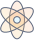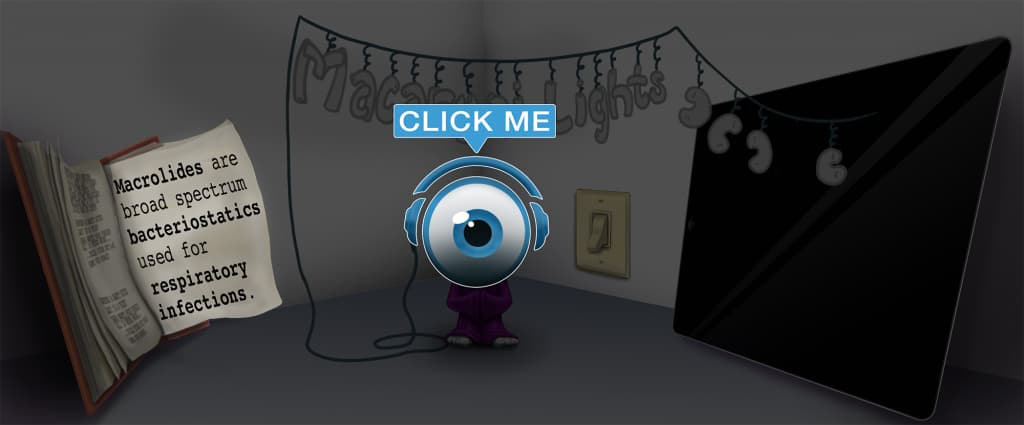# Master Alveolar Gas Equation with Picmonic for Medicine

## With Picmonic, facts become pictures. We've taken what the science shows - image mnemonics work - but we've boosted the effectiveness by building and associating memorable characters, interesting audio stories, and built-in quizzing.

### Alveolar Gas Equation

Ravioli Gas EquationPicmonic
The alveolar gas equation is used to calculate the alveolar partial pressure of oxygen (PAO2). This is done by the partial pressure of oxygen in the inspired air subtracted by the partial pressure of carbon dioxide in the arteries first divided by the respiratory quotient. The partial pressure of carbon dioxide divided by the respiratory quotient yields an estimate of the proportion of oxygen consumed by the body. Subtracting the partial pressure of oxygen in the air by the partial pressure of oxygen consumed by the body gives you the partial pressure of oxygen in the alveoli. The equation can be estimated as PAO2 = 150 - (PaCO2/0.8). It is important to calculate the PAO2 because this value is necessary in the calculation of alveolar-arterial gradient (A-a gradient), which is used clinically to diagnose the source of hypoxemia. The A-a gradient can be calculated by subtracting the alveolar partial pressure of oxygen from the arterial partial pressure of oxygen, which is normally 10 to 15 mmHg. Increased A-a gradient suggests possible shunting, VQ mismatch or fibrosis.
7 KEY FACTS
EQUATION
Partial Pressure of Alveolar Oxygen (PAO2)
Partial Pressure-gauge with Ravioli O2-tank

This is the partial pressure of oxygen (PO2) in the pulmonary alveoli, typically measured in millimeter of mercury (mmHg). This value is important for the calculation of other clinically relevant values, such as the A-a gradient.

Partial Pressure of Oxygen in the Inspired Air (PIO2)
Partial Pressure-gauge Spiral-straw O2

Oxygen exerts a partial pressure, which is determined by the environmental pressure. At sea level, the atmospheric pressure is 760 mmHg, and oxygen makes up 21 percent (20.094 percent to be exact) of inspired air. Since alveolar gas is assumed to be saturated with water vapor, the vapor pressure of water (~ 47 mmHg) must be subtracted from atmospheric pressure (760 - 47 = 713). So oxygen exerts a partial pressure of 713 x 0.21 = 150 mmHg.

PIO2 Normally Approximated = 150 mmHg
150 O2-tank

PIO2 in normal atmospheric settings is approximated as 150 mmHg.

Arterial Partial Pressure of CO2 (PaCO2)
Partial Pressure-gauge Artery-archer with CO2

The arterial carbon dioxide partial pressure (PaCO2) is an indicator of carbon dioxide in the blood. A high PaCO2, or hypercapnia, is indicative of underventilation, or more rarely, hypermetabolic activity. A low PaCO2, or hypocapnia, is indicative of hyperventilation. This value can be obtained clinically via an arterial blood gas measurement (ABG).

Respiratory Quotient (R)
Respirator Quotes

The respiratory quotient is the amount of CO2 produced, divided by the amount of O2 consumed. This varies from organism to organism, but can be approximated at 0.8 for humans.

R Normally Approximated = 0.8
Point (8) Ball

Respiratory quotient is approximately 0.8 in humans.

PAO2 = PIO2 - (PaCO2/R)
Ravioli with O2-tank EQUALS Spiral-straw with O2-tank SUB-tract Artery-Archer CO2 OVER Respirator Quotes

This is the equation used to calculate the alveolar partial pressure of oxygen. This equation can normally be approximated as PAO2 = 150 - (PaCO2/0.8).

Unlock all 7 facts & definitions with Picmonic Free!

## Take the Alveolar Gas Equation Quiz

Picmonic's rapid review multiple-choice quiz allows you to assess your knowledge.

## Picmonic for Medicine CoversMedicine (MD/DO)
1,800+ Videos
21,000+ Facts

## Our Story Mnemonics Increase Mastery and Retention

Memorize facts with phonetic mnemonics

Unforgettable characters with concise but impactful videos (2-4 min each)

### Ace Your Medicine (MD/DO) Classes & Exams with Picmonic:

#### Over 1,240,000 students use Picmonic’s picture mnemonics to improve knowledge, retention, and exam performance.